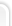## Большая коллекция рефератов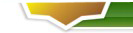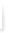### Реклама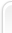# Physical Methods of Speed-Independent Module Design

Physical Methods of Speed-Independent Module Design

Oleg Izosimov

INTEC Ltd, Room 321, 7a Myagi Street, Samara 443093, Russia

1. Introduction

Any method of logic circuit design is based on using formal models

of gates and wires. The simplest model of a gate is determined by only two

"parameters": (a) Boolean function is to be calculated, (b) fixed

propagation delay. The simplest model of a wire is an ideal medium with

zero resistance and consequently, with zero delay. Such simple models allow

circuit design procedures which are a sequence of elementary steps easily

realized by a computer.

When logic circuits designed by using the simplest models expose

unreliable operation as in the case of gate delay variations, designers

introduce less convenient but more realistic models with arbitrary but

finite delay. Using more complicated models may produce logic circuits that

are called speed-independent .

In speed-independent circuits transition duration can be arbitrary.

So a centralized clock cannot be used. Instead special circuitry to detect

output validity is applied. Besides, additional interface circuitry is

needed to communicate with the environment in a handshaking manner. A speed-

independent circuit can be seen as a module consisting of combinational

logic (CL) proper, CL output validity detector (OVD) and interface

circuitry (Fig.1). To enable OVD to distinguish valid output data from

invalid ones, the redundant coding scheme was proposed . The main idea

of the scheme is to enumerate all possible input and output data, both

valid and invalid. The OVD must be provided with appropriate information on

data validity. To realize the idea of redundant coding some constraints on

CL design are imposed :

[pic]

(i) CL must be free of delay hazards, i.e. CL output data word must not

be dependent on the relative delay of signal paths through CL.

(ii) In changing between input states, any intermediate or transient

states that are passed through must not be mapped by CL onto valid output

states.

When these constraints were formulated, the circuit designers

realised that not every Boolean description could be implemented in a speed-

independent style. Other approaches to speed-independent module design were

needed.

SIM design as a science has two branches: logical and physical. For

a long time physical branch was overshadowed in spite of its

competitiveness. The main properties of physical approach to SIM design

are:

(a) Arbitrary coding scheme.

(b) Conventional procedure of operational unit design.

(c) Races of signals in SIM do not affect on its proper operation.

In this paper we propose an approach based on the physical nature of

transitions in CL. We believe that each transition is actually a transfer

of energy which can be naturally detected by physical methods.

From the viewpoint of a radio engineer CL behaves like a radio

transmitter. It emits radio frequencies in the 108-1010Hz band modulated by

signals of 106-108Hz. Obviously, the carrier wave is produced by gate

switchings during transitions in CL. The modulating wave is produced by

control schemes (OVD and interface circuitry) that detect transition

completion and inform the environment about the readiness of CL. OVD is a

kind of radio receiver that extracts the modulation envelope and enhances

the received signal. The main properties that OVD circuit must expose from

a radio engineer's point of view are selectivity and high gain. Since the

useful signals can propagate through non-conducting medium, OVD circuits

can be coupled with CL indirectly.

Advances in semiconductor technology gave birth to two methods of

transition detecting based on two kinds of the information carrying signal,

namely electromagnetic radiation and current consumption. Frequency of the

signal produced by switching logic gates is determined by gate delay.

For instance, CMOS network of 1-ns gates produces 1-GHz signal, ECL

array of 100-ps gates gives 10-GHz radiation. Logic circuits consisting of

10-ps gates will emit infra-red radiation. That signal could be easily

detected by photosensitive devices.

2. Background

Let us have a closer look at the structure of speed-independent

modules (SIM) as presented in Fig.1. All input data are processed in CL,

all output data are obtained from CL, too. So, CL is the only unit in SIM

which is involved in proper data processing. The result of that processing

is specified by Boolean functions. Algorithms for calculating the Boolean

function are realised by the internal structure of CL. Generally, its

structure is series-parallel as well as algorithm implemented.

When n-bit data word is put into the CL, n or more signal

propagation paths (SPPs) can be activated concurrently. So, one can say

that the calculation of a Boolean function by CL is of parallel nature. On

the other hand, each SPP is a gate chain which processes data in a serial

manner. So, calculation in CL is also of sequential nature.

The OVD circuit is intended for detecting transient and steady

"states" of CL. If any SPP in CL is still "active", CL is in transient

state, otherwise it is in steady state. Each gate switching results in both

logical and electromagnetic effects on its surrounding medium. The logical

effects of switching has been heavily investigated; we consider physical

one.

To provide speed-independence of the module the OVD and interface

circuitry must also work in a speed-independent mode. This means that any

arbitrary but finite transistor or wire delay cannot impair proper

operation of OVD and interface circuitry.

The interface circuitry is a mediator between OVD and environment of

SIM. It implements any kind of signalling convention, commonly a two- or

four-cycle one  based on request Req and acknowledgement Ack signal

using. The interface circuitry receives the output validity (OV) signal

from the OVD circuit, a Req signal from the environment and transmits an

Ack signal to the environment (Fig.1).

Consider an algorithm of operation for interface circuitry realizing

speed-independent four-cycle signalling convention (FCSC). In accordance

with FCSC the control signals must go in the following sequence: Req+OV-

Ack+Req-Ack- where "+" corresponds to rising the signal and "-" corresponds

to falling the signal. All signals are assumed to adhere to positive logic.

Initially the signals Req and Ack are low, the signal OV is high. If the

environment state changes, the Req signal rises and transient state of CL

occurs (OV-). Upon completion of the transitions in CL, signal OV rises and

the interface circuitry generates the Ack signal rising. After that the

environment produces a falling Req signal and then the interface circuitry

transmits the falling Ack signal to the environment. All the signals have

to be reset into the initial state.

To develop the interface circuitry a circuit designer must take into

account that any OVD circuit has finite (non-zero) turn-on delay ton. This

means that OVD cannot respond on transitions of short duration t tr< ton .

An example of interface circuitry is shown in Fig.2. It contains a

flip-flop, a NOR-gate, an asymmetrical delay and an inverter as an output

stage .

[pic]

The asymmetrical delay is intended for delaying Req rising signal

for + period where + > ton . Delaying Req falling signal noted - is to be

as short as possible. Note that speed-independent operation of interface

circuitry is vulnerable to delay + variation. If + becomes less than ton ,

proper operation of SIM can not be guaranteed. Otherwise, if + is much

more than ton , performance of SIM will be significantly reduced. To

provide exact accordance of + and ton a circuit emulator can be used.

Such an emulator is either an exact copy of OVD or its functional

copy, i.e. resistive-capacitive model of OVD's critical path. In the chip

the emulator must be placed next to active OVD circuit in order to ensure

identical conditions of fabrication and operation.

In this example we use a simplified asymmetrical delay implemented

as an asymmetrical CMOS inverter chain (Fig.3). Contrary to the common

inverter an asymmetrical one has non-equal rise and fall times of output

signal.

[pic]

A time diagram for interface circuitry is presented in Fig.4 for two

cases: (a) ttr < ton and (b) ttr ton. In case (a) the signal sequence

Req+Ack+ is formed for (++tNOR) period where tNOR is a NOR-gate delay. In

case (b) the above sequence is formed for (ttr +toff+tNOR) duration where

toff is a turn-off delay of OVD circuit. When the SIM returns to the

initial steady state, the signal sequence Req-Ack- is formed for (-+tNOR)

interval.

[pic]

After considering the SIM in operation it is obvious that the main

problems of the module design are in the area of CL and OVD interaction.

This includes (a) kind of signal used as a carrier of information about CL

output validity, and (b) method of OVD circuit design.

4. Current consumption detection

Using current consumption of CMOS CL for output validity detection

was proposed in 1990 . Contrary to the method of EMR detection this one

is based on introducing direct coupling of source and receiver. While CL is

in steady state it consumes current of about 10-9-10-8A which does not

allow OVD switching. The interface circuitry gets information on CL output

validity and in turn informs the environment about CL readiness to input

data processing. When an input data arrives CL changes its state to

"transient", current consumption increases to 10-4-10-2A, which switches

the OVD, thus informing the interface circuitry about output invalidity.

The latter lets the environment know about CL business.

After the computations in the CL are finished, the current consumption

decreases down to the steady state value, and the OVD sends a signal of

output validity.

4.1 Information carrying signal

Current consumption by CMOS CL contains useful information on CL

state. CMOS CL is a network of CMOS gates, so the current consumed by CL is

a superposition of currents consumed by CMOS gates included in the CL.

Each CMOS gate contains PMOS transistor and NMOS transistor networks

(Fig.5). While a gate is in a steady state either the PMOS or the NMOS

network is in a conducting mode. When a gate switches the non-conducting

transistor network becomes conducting. There is usually a short period in

switching time when both networks are in a conducting mode.

[pic]

Generally, current consumed by a CMOS gate includes three

components [9,10]:

(a) leakage current Ilk passing between power supply and ground due

to finite resistance of non-conducting transistor network;

(b) short-circuit current Isc flowing while both networks are in a

conducting mode;

(c) load capacitance CL charge current ILC flowing while a CMOS gate

is switching from low to high output voltage via conducting PMOS network

and CL .

SPICE simulation has shown  that amplitude of current consumed by

a typical CMOS inverter depends on CL and is limited by the non-zero

resistance of the conducting PMOS network (Fig.7). The integral of consumed

current is proportional to CL . When a gate switches from high to low

output voltage, the component ILC is negative by direction and negligible

by value (Fig.7b). It is evident, the switchings from high to low output

voltage occur at the expense of energy accumulated in CL during the

previous switching from low to high output voltage. The component Isc does

not depend on direction in which a gate switches.

[pic]

[pic]

The component ILC equals to ILC = CLVdd f where Vdd is a power

supply voltage, f is a gate switching frequency. Veendrick has investigated

the component Isc dependencies on CL and rise-fall time of input potential

signal . He showed that if both input and output signal have the same

rise-fall time, the component Isc cannot be more than 20 percent of summary

current consumption . However, when the output signal rise-fall time is

less than input one, the component Isc can be of the same order of

magnitude as ILC. In that case it must be taken into account. As to the

component Ilk, it entirely depends on CMOS process parameters and for state

of the art CMOS devices Ilk is about 10-15 -10-12 A.

So, the analysis of CMOS gate current consumption allows us to

conclude that in transient state a CMOS gate consumes a current I=

Ilk+Isc+ILC and in steady state it consumes only IlkVref, the

comparator output signal equals to logical "one" which means that the

outputs are invalid.

As it follows from the OVD circuit configuration,

[pic] [pic]

where Vdd is a voltage of power supply.

Equations (4) and (5) allow us to calculate the threshold voltage

drop V of the CVC circuit:

since [pic], so [pic] [pic]

If 0750mV, the diode D1 is in active mode and while rb 20, the component under

the sign of summation in Equation (7) can be much larger than the

component Cin. Due to voltage drop V the effective power supply voltage is

reduced and CL performance is decreased by about 35 percent .

In order to make SIM operating faster special attention must be paid

to reducing the capacitance introduced by CL.

4.3 Speed-independent address bus

The simplest case of CL is a scheme degenerated into a set of wires

called a multi-bit bus. Let us develop the OVD circuit for such a CL.

Multi-bit bus consists of several lines. Each line can be

considered as a medium for signal propagating from one end of the chip

to another. Delay of signal propagation through a line depends on several

factors:

(a) output impedance and symmetry of driver circuit;

(b) initial state of the line: if driver is symmetrical, line switching

from high to low voltage lasts shorter than reverse switching;

(c) electrical properties of the line as a signal propagation medium

(resistance of conducting layer and capacitances between the line and other

wires next to it);

(d) length of the line;

(e) input impedance and sensitivity of receiving circuit.

Since different lines of the bus operate in different conditions

(a)-(e), signal propagation delays are different, too. From the standpoint

of environment the bus behaves like any other more complicated CL.

Asynchronous RAM designers use a bus transition detector since 1980s

[13-15]. Such a detector is usually based on double-rail address coding

and two series connected transistors for each address bit . One of

the transistors receives the true address signal and the other receives

the complementary address signal of the particular address bit. For any

steady state condition one of the transistors will be turned on and one

will be turned off. There will be a finite rise and fall time during a

transition of the address bit. There is a short time during which both

transistors are conducting. The establishment of the conductive path

provides the detection of the address transition. In the first

asynchronous RAMs the output signal of the transition detector is used for

bit line precharging and for enabling/disabling sense amplifiers and

peripheral circuitry.

Self-timed RAM announced in 1983  used transition detectors not for

address transition only but also for detecting read/write completion and

address/bit line precharge completion as well.

The CMOS transition detector was invented in 1986 . This circuit

is also based on double-rail coding and uses a pair of series-connected

NMOS transistors (Fig.12). The scheme for n-bit bus control contains n line

transition detectors (LTDs) and n AND-gates. Outputs of AND-gates are

united in node M forming wired OR. The output inverter serves as a pulse

shaper. Capacitors C1 and C2 are intended to prolong rise time of the LTD

output signal (true and complementary). This is necessary for reliable

detection.

[pic]

The main drawback of the circuit is speed dependence. One can see

that if true and complementary address bit signal have different

propagation delays, the conducting path via NMOS transistors will never be

formed.

Using the OVD circuit proposed in Section 4.2 as LTD we can avoid

this drawback.

Note that address transmission through the address bus is

unidirectional. So to detect completion of bus transition it is enough to

recognize the bus state at the destination end. For this purpose we modify

CL to consist of n lines. The modification means introducing n LTDs, each

actually a CMOS inverter chain. Each chain contains two inverters loaded

with a capacitance (Fig.13). Input of each LTD is connected with

corresponding line of the bus at the destination end. Power supply pads of

all LTDs are connected to the current input of the same OVD circuit.

[pic]

The parameters of the input current signal for the OVD circuit are

varied by

(i) value of capacitances C1 and C2 ;

(ii) dimensions of MOS transistors M1 -M4 .

Since all transitions in CL are of the same duration and can be

lengthened to be outlast the OVD turning-on time, we simplify the

interface circuitry by disallowing the asymmetrical delay.

Due to short duration of normal transition in this CL we must take

into account the integral nature of the sensitivity of the OVD circuit. OVD

sensitivity depends on both amplitude and width of input current pulse.

Simulated operation region of the OVD circuit for current pulses shorter

than 30ns is shown in Fig.14. It is obvious that in this case the threshold

of the OVD circuit must be determined by threshold charge Qth value. The

OVD input charge Q equals to [pic] where I is OVD input current, t is a

moment of time when transition occurs, w is a width of input current

pulse. Turning-on condition for the OVD circuit is Q=Qth.

[pic]

When the LTD circuit shown in Fig.13 is used, the charge value Q is

determined by either C1 or C2. Namely, if the line goes from low to high

voltage, Q=VC2. If the line goes in the reverse direction then [pic] where

V is charging/discharging voltage, approximately equal to the effective

power supply voltage: VVdd -V. Here Vdd is OVD power supply voltage and V

is CVC voltage drop.

The OVD circuit with typical parameters (See Table 1) has a

threshold charge value Qth =4.010-12 C. When C1 =C2 =CL , the minimal value

of CL providing OVD capacity for operation is about 1.010-12 F.

Influence of transistors M1 -M4 dimensions on LTD delay d is

determined by approximation :

[pic]

where ~ is a sign of proportionality, Gn and Gp are the conductances of

NMOS and PMOS transistors respectively (CL =C1 =C2.)

Since [pic] and [pic] where W and L are width and length of

transistor channels of the corresponding conduction type, the LTD delay d

is proportional to [pic].

It has been obtained that for [pic], [pic], CL=1.0pF and Vdd-V=5.0V

the LTD delay d=7.6ns.

When LTD works jointly with the OVD in the speed-independent bus,

the real value of the LTD delay will increase by 30-40 percent due to

OVD's R1 effect on the effective power supply voltage.

To determine the appropriate value of R1 in the OVD circuit we must

know threshold input current Ith corresponding to threshold voltage drop

Vth recommended to be equal to 400mV.

Average input current Iav in transient state of one line is

determined by the expression Iav =CLv where v is the average rate of

increase in the output signal for an inverter included in LTD. For typical

values v=1.0109 Volts per second and CL =1.0pF, Iav =1.0mA. Accepting Ith

=0.4mA and Imax=2.0mA we obtain R1=1k and rb=100.

Simulation has shown that in this case OVD turning-on delay can be

approximated by an empirical expression:

ton[ns]=8.1+0.1n

where n is the address bus bit capacity. Total delay of recognizing address

transition ttot =dg+ton where g is a coefficient of the LTD delay increase

due to reducing power supply voltage. As we showed above g1.35. It can be

seen that if n=32, ttot=21.6ns.

The circuit we use in this Section as a CL was a touch-stone for

many speed-independent circuit designers for about four decades. We mean a

ripple carry adder (RCA) which is actually a chain of one-bit full adders

(Fig.14).

[pic]

Each full adder calculates two Boolean functions: sum si=aibici and

output carry ci+1=aibi+bici+aici where ai, bi are summands, ci is input

carry and stands for XOR operation.

In 1955 Gilchrist et al. proposed speed-independent RCA with carry

completion signal . In 1960s that circuit was carefully analyzed and

improved [19-21]. In 1980 Seitz used RCA for illustrating his concept of

equipotential region and his approach to self-timed system design .

Now we use RCA as a CL for illustrating our approach to SIM design.

As it was shown in Section 4.2 the turn-on and turn-off delays of

the OVD circuit are proportional to the equivalent capacitance Ceq

associated with OVD circuit input. Capacitance Ceq depends linearly on a

number of gates N in CMOS CL. To speed up a SIM it is necessary to reduce a

number N. This can be reached by structural decomposition CMOS CL into

subcircuits CL1, CL2, etc. Each subcircuit CLi is connected to its own

detecting circuit OVDi or directly to the power supply if this subcircuit

transition does not affect the transition duration in CL as a whole. Each

detecting circuit OVDi generates its own OV signal which is combined with

other OVDs' output signals via a multi-input OR (NOR) element. The output

signal of that element serves as OV signal of the CMOS CL.

Multi-bit RCA computation time is determined by length of maximal

activated carry chain. A lot of papers were devoted to analysis of carry

generation and carry propagation in RCA [19-21], many of them contained

their own methods for estimation or calculation of average maximal

activated carry chain. We do not intend to add another one.

Let us have a look inside RCA. As it was mentioned above RCA

consists of one-bit full adders and each full adder consists of two

parts: forming sum si part and forming carry ci+1 part (Fig.16).

In multi-bit RCA all forming sum parts do not interact with each

other and do not affect on transition duration in RCA. Each forming carry

ci+1 part receives ci signal from preceding forming carry part and sends

ci+1 signal to consequent one.

To decompose RCA we use three heuristic tricks:

(i) All forming sum parts we connect directly to power supply.

(ii) We divide each forming carry part into three subcircuits denoted in

Fig.16 by numbers 1,2 and 3. All subcircuits 1 we connect directly to

power supply because they do not contain input ci and so do not contain

carry propagation path.

(iii) All subcircuits 2 we connect to OVD1 and all subcircuits 3 we

connect to OVD2. Outputs of OVD1 and OVD2 are connected to two-input

NOR-gate forming RCA OV signal in positive logic manner (Fig.17).

OVD1 and OVD2 input currents I1 and I2 curves for 6-bit RCA and

longest transition duration are shown in Fig.18.

Accepting Vth1,2=400mV we calculated the OVD circuits parameters. It

was obtained R11=5k, Ith1=0.08mA, R12=3k, Ith2=0.13mA. OVD1 and OVD2 delay

dependencies on a number of bits in RCA are shown in Fig.19.

4.5 Comparison of SIMs with synchronous counterparts

Transition duration in CL is a random variable. Probability of

transition with duration D is determined by implemented Boolean function

and distribution of input logical combinations. Domain of possible values

for variable D occupies the interval [0;Dmax]. Here Dmax is a length of

critical path in CL.

Let [pic] is a mathematical expectation of transition duration in CL

where Di is a length of i-th SPP in CL, pi is a probability of i-th path

being the longest activated SPP.

When CL works in the synchronous mode, the cycle duration Ts is

chosen with regard to maximal transition duration Dmax. Certain margin must

be added to Dmax to provide reliable operation of CL in the case of CL

parameter variations: Ts =kDmax where k is a margin coefficient.

In SIM cycle duration is a random variable with expectation Tsi =

gDme+toff+tif where g is a coefficient of CL delay increasing due to

reducing power supply voltage, toff is turn-off delay of the OVD circuit,

tif is an interface circuitry delay.

We determine efficiency E for speed-independent mode of CL operation

as relative increase of SIM performance in comparison to its synchronous

counterpart:[pic].

Generally, speed-independent mode is more efficient than synchronous

one if Ts >Tsi or, in other words, [pic].

In the case of RCA [pic] where tc is a delay of carry forming part,

n is a number of full adders in RCA.

It has been shown  that in n-bit RCA Dme tclog2(5n/4). Then, in

the case of speed-independent operation Tsi=gtclog2(5n/4)+toff+tif.

We have obtained dependencies of Ts , Tsi on a number of bits in

RCA that are shown in Fig.20. As it can be seen, speed-independent

operation of RCA is more efficient while n>8.

5.Conclusion

6.Acknowledgement

I would like to thank Igor Shagurin and Vlad Tsylyov of the Moscow

Physical Engineering Institute for helpful discussions of this work. I am

also grateful to Chris Jesshope of University of Surrey and Mark Josephs of

Oxford University who kindly provided the latest material on their research

in the area of delay-insensitive circuit design.

References

 Miller, R.E., Switching theory (Wiley, New York, 1965),

vol.2, Chapter 10.

 Unger, S.H., Asynchronous Sequential Switching Circuits

(Wiley, New York, 1969).

 Armstrong, D.B., A.D. Friedman, and P.R. Menon, Design of

Asynchronous Circuits Assuming Unbounded Gate Delays, IEEE

Trans.on Computers C-18 (12) (1969) 1110-1120.

 Seitz, C.L., System timing, in: C.A. Mead and L.A. Conway,

eds., Introduction to VLSI Systems (Addison-Wesley, New

York, 1980), Chapter 7.

 Izosimov, O.A., I.I. Shagurin, and V.V. Tsylyov, Physical

approach to CMOS module self-timing, Electronics Letters 26 (22)

(1990) 1835-1836.

 Veendrick, H.J.M., Short-circuit dissipation of static CMOS

circuit and its impact on the design of buffer circuits,

IEEE J. Solid-State Circuits SC-19 (4) (1984) 468-473.

 Chappell, B.A, T.I. Chappell, S.E. Schuster, H.M. Segmuller,

J.W. Allan, R.L. Franch, and P.J. Restle, Fast CMOS ECL

receivers with 100-mV worst-case sensitivity, IEEE J. Solid-State

Circuits SC-23 (1) (1988) 59-67.

 Chu, S.T., J. Dikken, C.D. Hartgring, F.J. List, J.G.

Raemaekers, S.A. Bell, B. Walsh, and R.H.W. Salters, A 25-ns

Low-Power Full-CMOS 1-Mbit (128K8) SRAM, IEEE J. Solid-State

Circuits SC-23 (5) (1988) 1078-1084.

 Frank, E.H., and R.F. Sproull, A Self-Timed Static RAM, in:

Proc. Third Caltech VLSI Conference (Springer-Verlag,

Berlin, 1983) pp.275-285.

 Donoghue, W.J., and G.E. Noufer, Circuit for address transition

detection, US Patent 4563599, 1986.

 Huang, J.S.T., and J.W. Schrankler, Switching characteristics

of scaled CMOS circuits at 77K, IEEE Trans. on Electron

Devices ED-34 (1) (1987) 101-106.

 Gilchrist, B., J.H. Pomerene, and S.Y. Wong, Fast Carry Logic

for Digital Computers, IRE Trans. on Electronic Computers EC-4

(4) (1955) 133-136.

 Hendrickson, H.C., Fast High-Accuracy Binary Parallel

Addition, IRE Trans. on Electronic Computers EC-9 (4) (1960)

465-469.

 Majerski, S., and M. Wiweger, NOR-Gate Binary Adder with Carry

Completion Detection, IEEE Trans. on Electronic Computers EC-16

(1) (1967) 90-92.

 Reitwiesner, G.W., The determination of carry propagation

length for binary addition, IRE Trans. on Electronic Computers

EC-9 (1) (1960) 35-38.

Appendix

SPICE2G.6: MOSFET model parameters

| | | | | | |

| | | | |VALUE | |

| |Name |Parameter |Units |PMOS |NMOS |

|1 |level |model index |- |3 |3 |

|2 |VTO |ZERO-BIAS THRESHOLD VOLTAGE |V |-1.337 |1.161 |

|3 |KP |TRANSCONDUCTANCE | | | |

| | |PARAMETER |A/V2 |2.310-5 |4.610-5 |

|4 |GAMMA |BULK THRESHOLD PARAMETER |[pic] |0.501 |0.354 |

|5 |PHI |SURFACE POTENTIAL |V |0.695 |0.660 |

|6 |RD |DRAIN OHMIC RESISTANCE |OHM |333 |85 |

|7 |RS |SOURCE OHMIC RESISTANCE |OHM |333 |85 |

|8 |CBD |ZERO-BIAS B-D JUNCTION | | | |

| | |CAPACITANCE |F |1.9810-14|6.910-15 |

|9 |CBS |ZERO-BIAS B-S JUNCTION | | | |

| | |CAPACITANCE |F |1.9810-14|6.910-15 |

|10|IS |BULK JUNCTION SATURATION | | | |

| | |CURRENT |A |3.4710-15|9.2210-15|

|11|PB |BULK JUNCTION POTENTIAL |V |0.8 |0.8 |

|12|CGSO |GATE-SOURCE OVERLAP CAPACI- | | | |

| | |TANCE PER METER CHANNEL WIDTH|F/M |6.7010-10|3.3010-10|

|13|CGDO |GATE-DRAIN OVERLAP CAPACI- | | | |

| | |TANCE PER METER CHANNEL WIDTH|F/M |6.7010-10|3.3010-10|

|14|CGBO |GATE-BULK OVERLAP CAPACITANCE| | | |

| | | |F/M |1.9010-9 |2.6010-9 |

| | |PER METER CHANNEL LENGTH | | | |

|15|RSH |DRAIN AND SOURCE DIFFUSION | | | |

| | |SHEET RESISTANCE |OHM/SQ|55 |30 |

|16|CJ |ZERO-BIAS BULK JUNCTION | | | |

| | |BOTTOM | | | |

| | |CAPACITANCE PER SQ METER OF |F/M2 |3.5310-4 |1.2410-4 |

| | |JUNCTION AREA | | | |

|17|MJ |BULK JUNCTION BOTTOM GRADING | | | |

| | |COEFFICIENT |- |0.5 |0.5 |

|18|CJSW |ZERO-BIAS BULK JUNCTION SIDE-| | | |

| | | | | | |

| | |WALL CAPACITANCE PER METER OF|F/M |1.7110-10|3.2010-11|

| | | | | | |

| | |JUNCTION PERIMETER | | | |

| | | | | | |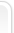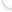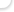### Опросы

Оцените наш сайт?
 Хорошо Отлично Полезно было посетить Добавил в закладки Еще зайду не раз Теперь я здесь постоянный

### Кто на сайте?

Сейчас на сайте находятся:
345 гостейВсе права защищены © 2010